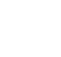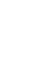# Interactive AMD Athlon 64 Socket AM2 CPU Pinmod guide

##### Original CPU Vcore selection 0,800 Volt 0,825 Volt 0,850 Volt 0,875 Volt 0,900 Volt 0,925 Volt 0,950 Volt 0,975 Volt 1,000 Volt 1,025 Volt 1,050 Volt 1,075 Volt 1,100 Volt 1,125 Volt 1,150 Volt 1,175 Volt 1,200 Volt 1,225 Volt 1,250 Volt 1,275 Volt 1,300 Volt 1,325 Volt 1,350 Volt 1,375 Volt 1,400 Volt 1,425 Volt 1,450 Volt 1,475 Volt 1,500 Volt 1,525 Volt 1,550 Volt Select the desired Vcore 0,800 Volt 0,825 Volt 0,850 Volt 0,875 Volt 0,900 Volt 0,925 Volt 0,950 Volt 0,975 Volt 1,000 Volt 1,025 Volt 1,050 Volt 1,075 Volt 1,100 Volt 1,125 Volt 1,150 Volt 1,175 Volt 1,200 Volt 1,225 Volt 1,250 Volt 1,275 Volt 1,300 Volt 1,325 Volt 1,350 Volt 1,375 Volt 1,400 Volt 1,425 Volt 1,450 Volt 1,475 Volt 1,500 Volt 1,525 Volt 1,550 Volt